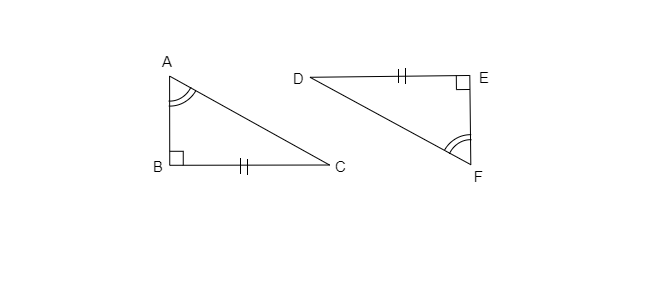# Explain, why $‚àÜABC ‚âÖ ‚àÜFED$."

Given: Two triangles $‚àÜABC$ and $‚àÜFED$.

To do: To explain that $‚àÜABC ≅ ‚àÜFED$.

Solution:

Given $\angle A\cong\angle F$

$BC=ED$

$\angle B=\angle E$

In $\triangle ABC$ and $\triangle FED$

$\angle B=\angle E=90^{\circ}$

$\angle A=\angle F$

$BC=ED$

Therefore, $\triangle ABC\cong\triangle FED$          [RHS congruence rule]